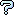All about flooble | fun stuff | Get a free chatterbox | Free JavaScript | Avatarsperplexus dot infoFurther Arithmetic Integers (Posted on 2006-06-27)Consider three positive integers x < y < z in arithmetic sequence, and determine all possible solutions of: x4 + y4 = z4 - 64

 Submitted by K Sengupta Rating: 2.5000 (2 votes) Solution: (Hide) (x,y,z) = (1, 2, 3) and (7, 8, 9) constitutes the only possible solution to the given equation. EXPLANATION: By the conditions of the problem: z = y + a and x = y �a , where a is any positive integer less than x. Accordingly, in terms of provisions of the problem under reference: (y + a)^4 - 64 = (y - a)^ 4 + y^4 or, 8*(y^3)* a + 8*(a^3)*y = y^4 + 64-------(#) It is clearly observed from (#) that y is even ,so that substituting y = 2*p , we obtain : 4*(p^3)*a + (a^3)*p - 4 = p^4 Or, p(4*(p^2)*a + a^3 - p^3) = 4; so that p must be a positive divisor of 4 giving p= 1,2,4 Now; p=1 gives, a^3 + 4*a - 5 = 0, which yields:(a-1)((a^2)+ a + 5) =0; giving a = 1, (-1 +/- sqrt(-19))/2, so that the only integral root in this case is given by a = 1 Hence, y=2*p = 2 Consequently, z= y+1 = 3 and x = y -1 = 1 p=2 gives, (a^3) + 16*a - 10 = 0, which does not possess any positive integer solution in a. Now; p=4 gives, 4*(a^3) + 256*a - 4 = 256 or, (a^3) + 64*a - 65 = 0, giving: (a-1)*((a^2) +a + 65)= 0, giving a=1 or, a= (-1+/- sqrt(-16899))/2. Since, a is a positive integer, it follows that a=1; giving: y=2*p = 2*4 = 8.Consequently, z= y+1 = 9 and x = y -1 = 7 Therefore, (x, y, z) =(1, 2, 3) and (7, 8, 9) constitutes the only positive integral solutions to the equation under reference.--------------------------------------------------------- Also refer to Bractals' solution and explanation.Subject Author Dategenearl problem :-D Daniel 2006-06-28 22:53:13re(2): Solution Bractals 2006-06-28 10:53:32re(2): Solution Bractals 2006-06-28 10:32:38re: Solution Yosippavar 2006-06-28 07:21:43Not a Logical answer Yosippavar 2006-06-28 07:14:29mathematica solution (spoiler) Daniel 2006-06-28 06:03:32re: Solution Trevor 2006-06-27 21:37:31Solution Bractals 2006-06-27 21:01:09re: not a proof but may be spoiler Trevor 2006-06-27 16:16:56not a proof but may be spoiler Charlie 2006-06-27 16:02:16Please log in:

 Search: Search body:
Forums (1)